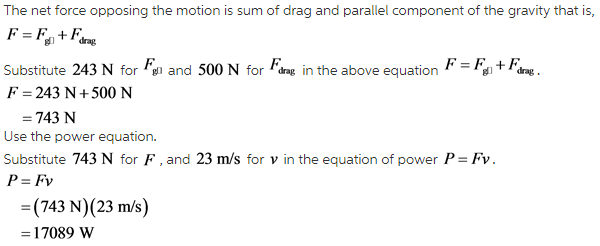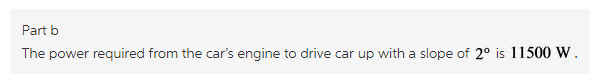# A 710kg car drives at a constant speed of 23m/s . It is subject to a drag force of 500 N

A 710kg car drives at a constant speed of 23m/s . It is subject to a drag force of 500 N. What power is required from the car’s engine to drive the car (a) on level ground? (b) up a hill with a slope of 2.0? ?

Concepts and reason
The concept required to solve this problem is power.
Initially use the equation of power due to force to calculate power required.
Finally calculate the net force in the opposite direction to the motion of car and substitute it in the power equation to calculate the power required from the engine.

Fundamentals
The power due to an applied force to keep an object at constant velocity is given as,

(a)
Use the equation of power.Explanation:
The force required to keep the car at constant velocity is the net drag force acting on the car.
Substitute the value of drag force and velocity in the equation of power to calculate the power required.

(b)
Use the equation of parallel component of gravity.Explanation:
The force required to keep the car at constant velocity is the sum of net drag force acting on the car and parallel component of the gravity.
Substitute the value of net force and velocity in the equation of power to calculate the power required.